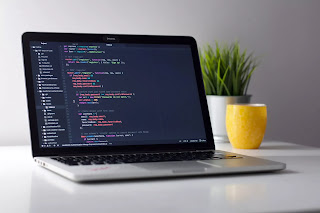## Positive AND codechef October long challenge Solution |Codechef October long challenge editorial -

This Problem is taken from Codechef  October long challenge and I have explained Positive and complete solution.

### Problem Statement-

A permutation ${p}_{1},{p}_{2}...{p}_{N}$ of $\left\{1,2,...,N\right\}$ is beautiful if ${p}_{i}\mathrm{&}{p}_{i+1}$ is greater than 0 for every $1\le i . You are given an integer $N$, and your task is toconstruct a beautiful permutation of length $N$ or determine that it's impossible.

Note that $a\mathrm{&}b$ denotes the bitwise AND of $a$ and $b$.

### Input:

First-line will contain $T$, number of testcases. Then the testcases follow. Each testcase contains a single line of input, an integer $N$.

### Output:

For each test case output $-1$ if there is no suitable permutation of length $N$, otherwise output $N$ integers in a single line which form a beautiful permutation. If there are multiple answers output any of them.

### Constraints

• $1\le N\le {10}^{5}$
• The sum of $N$ over all test cases does not exceed ${10}^{6}$

• 50 points : $1\le N,T\le 9$
• 50 points : Original constraints

### Sample Input:

3
4
3
5


### Sample Output:

-1
1 3 2
2 3 1 5 4## link of problem-https://www.codechef.com/OCT20B/problems/POSANDRead More-Chef and Easy Queries CodeChef October long challenge problem solution editorialread more-Covid Run Codechef October long challenge problem solution| Codechef October long challenge problem solutionread More-Replace for X codechef October long challenge solution | codechef October long challenge editorial

### Solution-

Positive AND problem was little bit tricky problem.
mistake 1- Do not try to build the same output as given in custom input.
mistake 2- these can more then one ans of this problem so you can print any one of them.

I was thinking while solving the problem what wrong with the given permutation why it is not valid solution.
so i got ans by the observation that when a 2^n(1,2,4,8,16....) number AND with 2^n-1(1,3,7,15) number it forms always zero because in 2^n number only MSB present(eg 2=10,4=100,8=1000) other bit are equals to zero.
So my assumed permutation can be valid answer if i swap 2^n number with there next elements. so that it can not form 0 while doing AND
operation.
To make my permutation valid I have to swap these numbers with to number next to it. For eg. I will swap 8 to 9,16 to 17 ,32 to 33...
and print -1 if N is 2^n because in this case we cannot swap N to N+1 because we have no choice left.

So here is my working code.